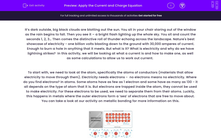# Apply the Current and Charge Equation

In this worksheet, students will look at the concept of current and charge and use these ideas to solve more complex problems.Key stage:  KS 4

Year:  GCSE

GCSE Boards:   AQA, AQA Trilogy, AQA Synergy, OCR 21st Century, OCR Gateway, Pearson Edexcel, Eduqas,

Popular topics:   Physics worksheets, Electricity worksheets

Difficulty level:#### Worksheet Overview

It’s dark outside, big black clouds are blotting out the sun. You sit in your chair staring out of the window as the rain begins to fall. Then you see it – a bright flash lighting up the whole sky. You sit and count the seconds 1, 2, 3… Then comes the distinctive roll of thunder echoing across the landscape. Nature's best showcase of electricity - one billion volts blasting down to the ground with 30,000 amperes of current. Enough to burn a hole in anything that it meets. But what is it? What is electricity and why do we have lightning strikes?  In this activity, we will be looking at what a current is and how to make one, as well as some calculations to allow us to work out current.

To start with, we need to look at the atom, specifically the atoms of conductors (materials that allow electricity to move through them). Electricity needs electrons -  no electrons means no electricity. Where do you find electrons? In atoms. Some atoms have as few as 1 electron and some have as many as 130 - it all depends on the type of atom that it is. But electrons are trapped inside the atom, they cannot be used to make electricity. For these electrons to be used, we need to separate them from their atoms. Luckily, this happens in metals where the outer electrons form a ‘sea’ of electrons that are free to move about. You can take a look at our activity on metallic bonding for more information on this.When you have some electrons that can move around, you then need to actually start moving them. This is where the idea of charge comes in and it is quite important here. Electrons are negatively charged and they are normally attracted to the positively charged nucleus of the atom (where the protons and neutrons live). This is because opposite charges attract each other. When we have removed electrons from their atoms, all we need to do is to place them near a positive charge and the electrons will flow towards that charge. The opposite is true as well - if you place a negative charge near to them, they will move away from that charge.

When these electrons are flowing, you have a current. The definition of current is ‘the number of electrons passing a point per second’ so the more electrons there are, the more current there is. Let’s think about lightning again. The electrons must have been free to move in the clouds – this happens because when ice particles collide with other ice particles, it frees up some electrons. There must have been either an opposite charge or a similar charge near them – both in this case - the ground is the opposite charge, pulling the electrons down and the cloud is the similar charge, pushing the electrons away. This then gives us enough energy to have a whole bunch of electrons moving from the cloud to the ground. That is your lightning strike.

Now, how many electrons are in a lightning strike? Well, we can work out the amount of charge by using the equation below, which is fondly known as the QUIT equation.

Q = It

Q = charge (measured in coulombs (C))
I = current (measured in amperes or amps (A))
t = time (measured in seconds (s))

Let’s use this equation in an example:

Question:  A wire has a charge of 4 C moving though it in 5 s. Calculate the current in the wire.

Step 1   Highlight all of the numbers in the equation:

A wire has a charge of 4 C moving though it in 5 s. Calculate the current in the wire.

Step 2   Write out the numbers next to their symbols:

Q = 4 C
I = ?
t = 5 s

Step 3   Rearrange the equation:

Q = It

I = Q ÷ t

Step 4   Put the numbers into the equation:

I = 4 ÷ 5

Step 5   Do the maths:

I = 0.8 A